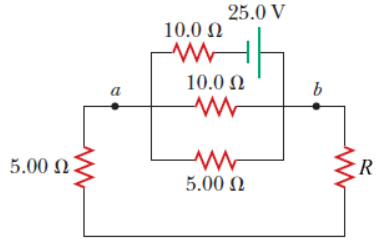# Problem: Consider the circuit shown in the figure below. (Let R = 36.0 Ω.)(a) Find the current in the 36.0-Ω resistor.A(b) Find the potential difference between points a and bV

###### FREE Expert Solution
95% (219 ratings)View Complete Written Solution
###### Problem Details

Consider the circuit shown in the figure below. (Let R = 36.0 Ω.)(a) Find the current in the 36.0-Ω resistor.

A

(b) Find the potential difference between points a and b

V